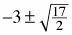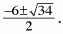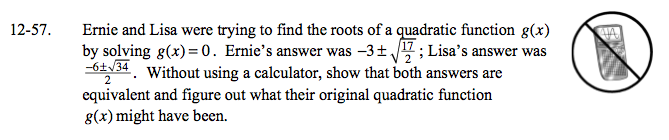### Home > A2C > Chapter 12 > Lesson 12.1.3 > Problem12-57

12-57.

Ernie and Lisa were trying to find the roots of a quadratic function g(x) by solving g(x) = 0. Ernie'sanswer was. Lisa's answer wasWithout using a calculator, show that both answers are equivalent and figure out what their original quadratic function g(x) might have been. Homework Help ✎